# Praxis I Math: Square Roots and Exponents Chapter Exam

Exam Instructions:

Choose your answers to the questions and click 'Next' to see the next set of questions. You can skip questions if you would like and come back to them later with the yellow "Go To First Skipped Question" button. When you have completed the practice exam, a green submit button will appear. Click it to see your results. Good luck!

### Page 1

#### Question 1 1. Simplify the expression using positive exponents.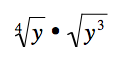#### Question 2 2. Using positive exponents, simplify the expression.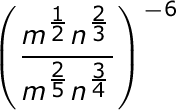#### Question 3 3. Simplify.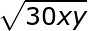### Page 2

#### Question 6 6. Simplify using ONLY positive exponents.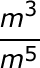#### Question 8 8. Simplify the expression below using only positive exponents.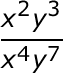#### Question 10 10. Which of the following is equivalent to the expression below?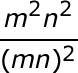### Page 3

#### Question 12 12. Simplify the expression below using positive exponents.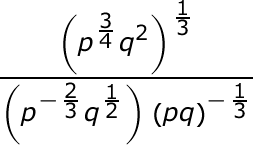#### Question 13 13. Simplify using only positive exponents.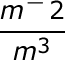#### Question 14 14. Simplify.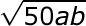### Page 4

#### Question 16 16. Which of the following is equivalent to the following expression?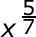#### Question 17 17. Simplify.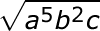#### Question 19 19. Simplify the expression below.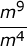#### Question 20 20. Which of the following would be true regarding the following exponential expression?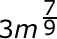### Page 5

#### Question 21 21. Which of the following radical expressions is equal to this exponential expression?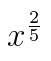#### Question 23 23. Simplify the expression below using positive exponents only.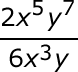#### Question 24 24. Simplify using ONLY positive exponents.### Page 6

#### Question 26 26. Which of the following exponential expressions is equal to this radical expression?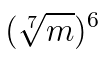#### Question 29 29. Which of the following is equivalent to the expression below?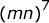#### Praxis I Math: Square Roots and Exponents Chapter Exam Instructions

Choose your answers to the questions and click 'Next' to see the next set of questions. You can skip questions if you would like and come back to them later with the yellow "Go To First Skipped Question" button. When you have completed the practice exam, a green submit button will appear. Click it to see your results. Good luck!

Support